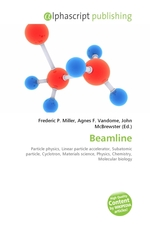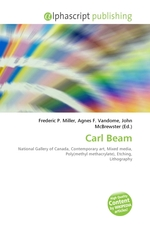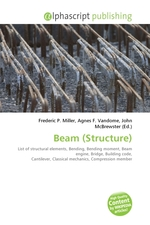# An Introduction to Mathematica

Warning: mysql_num_rows() expects parameter 1 to be resource, boolean given in /home/web/naiti.in/lib.php on line 113Автор Ramsden P., Kent P.
 Год 1999
 Страниц 41### Описание:

The course is delivered in two versions, for first year Civil and Chemical Engineering students. Course length is 6 hours and 10 hours, respectively. The course consists of "core" material on Mathematica, combined with specific engineering topics that differ in each department - for Civil, beams and vibrations, for Chemical, theory of gases and fluid flow. There are also some specific mathematical topics. The overall purpose of these courses is to show students that mathematical software like Mathematica is relevant to their studies. Eventually, we hope to strengthen this message by having Mathematica appear in lectures and assignments in other first-year courses. These courses have been described in a series of articles, all available online at http://metric.ma.ic.ac.uk/articlesTopics:Civil Engineering: Mathematica graphics and animations - static and quasi-static beam deflection problems Numerical solution of differential equations - damped and forced vibrations of a loaded beam Chemical Engineering: Mathematica graphics and animations - the Van der Waals gas equation, fluid flow around obstacles Mathematica symbolics - finding stationary points on curves and surfaces Mathematica numerics - calculating friction factors for flow in pipes, computing particle paths in fluids Simple programming in Mathematica"

### Похожие книгиNew Developments in Advanced WeldingГод: 2005X-Ray Scattering of Soft MatterАвтор: Stribeck N.Год: 2007Gazela BridgeГод: 2010BeamlineГод: 2011Carl BeamГод: 2011Beam (Structure)Год: 2011